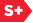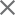### Proceedings Paper

Interpolatory fixed-point algorithm for an efficient computation of TE and TM modes in arbitrary 1D structures at oblique incidence
Author(s): Manuel Pérez Molina; Jorge Francés Monllor; Mariela Álvarez López; Cristian Neipp López; Luis Carretero López
Format Member Price Non-Member Price
PDF \$17.00 \$21.00

Paper Abstract

We develop the Interpolatory Fixed-Point Algorithm (IFPA) to compute efficiently the TE and TM reflectance and transmittance coefficients for arbitrary 1D structures at oblique incidence. For this purpose, we demonstrate that the semi-analytical solutions of the Helmholtz equation provided by the fixed-point method have a polynomial dependence on variables that are related to the essential electromagnetic parameters -incidence angle and wavelength-, which allows a drastic simplification of the required calculations taking the advantage of interpolation for a few parameter values. The first step to develop the IFPA consists of stating the Helmholtz equation and boundary conditions for TE and TM plane incident waves on a 1D finite slab with an arbitrary permittivity profile surrounded by two homogeneous media. The Helmholtz equation and boundary conditions are then transformed into a second-order initial value problem which is written in terms of transfer matrices. By applying the fixed-point method, the coefficients of such transfer matrices are obtained as polynomials on several variables that can be characterized by a reduced set of interpolating parameters. We apply the IFPA to specific examples of 1D diffraction gratings, optical rugate filters and quasi-periodic structures, for which precise solutions for the TE and TM modes are efficiently obtained by computing less than 20 interpolating parameters.

Paper Details

Date Published: 14 May 2010
PDF: 11 pages
Proc. SPIE 7717, Optical Modelling and Design, 771704 (14 May 2010); doi: 10.1117/12.854275
Show Author Affiliations
Manuel Pérez Molina, Univ. de Alicante (Spain)
Jorge Francés Monllor, Univ. de Alicante (Spain)
Mariela Álvarez López, Univ. de Alicante (Spain)
Cristian Neipp López, Univ. de Alicante (Spain)
Luis Carretero López, Univ. Miguel Hernández de Elche (Spain)

Published in SPIE Proceedings Vol. 7717:
Optical Modelling and Design
Frank Wyrowski; John T. Sheridan; Jani Tervo; Youri Meuret, Editor(s)